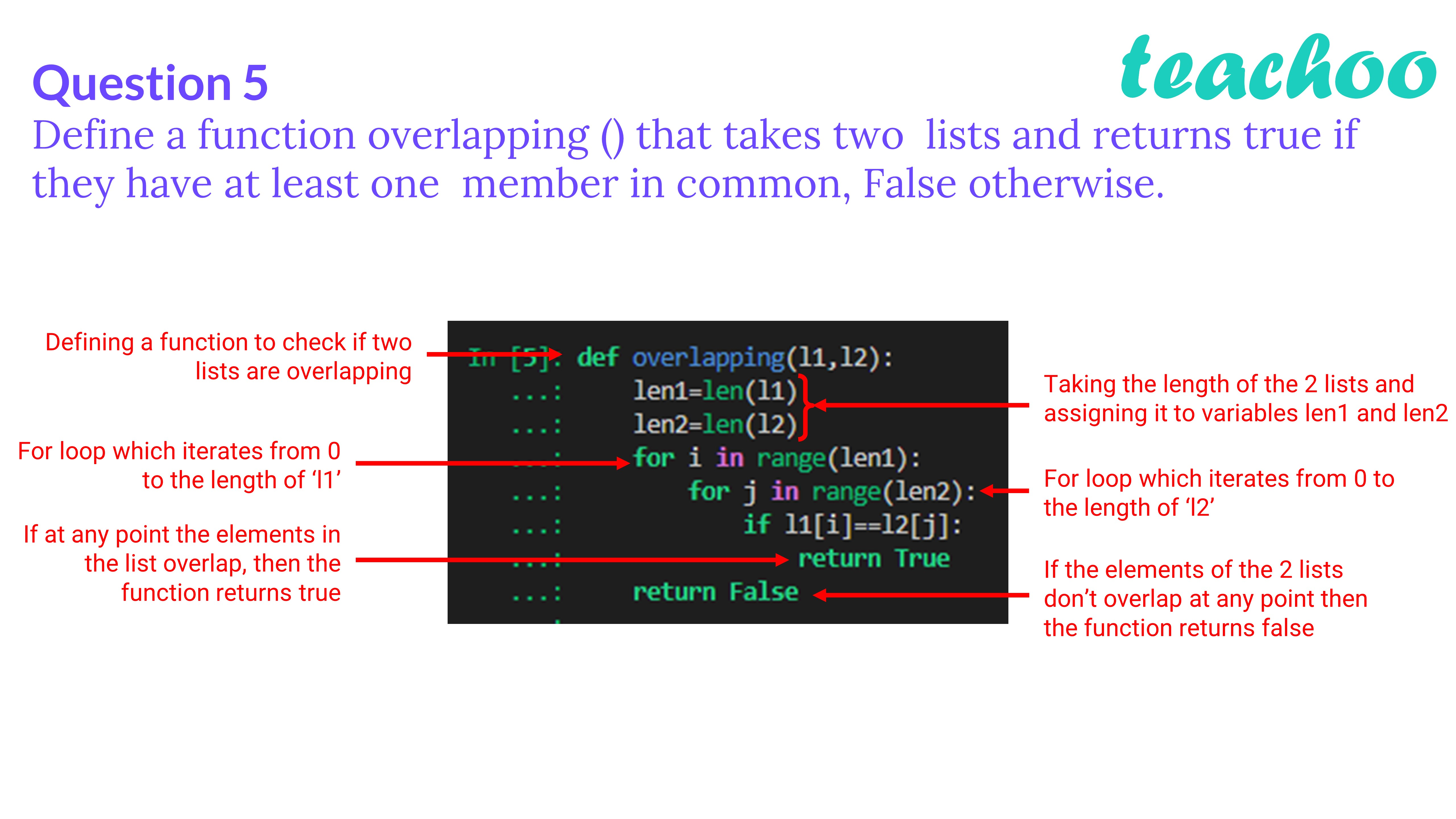Past Year - 3 Mark Questions

Computer Science - Class 12
Chapter 14 - Functions in python

## Define a function overlapping () that takes two lists and returns true if they have at least one member in common, False otherwise.

``` def overlapping (l1,l2): ```

```     len1=len(l1) ```

```     len2=len(l2) ```

```     for i in range(len1): ```

```         for j in range(len2): ```

```             if l1[i]==l2[j]: ```

```                 return True ```

```     return False ```Learn in your speed, with individual attention - Teachoo Maths 1-on-1 Class

### Transcript

Question 5 Define a function overlapping () that takes two lists and returns true if they have at least one member in common, False otherwise. Defining a function to check if two lists are overlapping For loop which iterates from 0 to the length of ‘l1’ If at any point the elements in the list overlap, then the function returns true Taking the length of the 2 lists and assigning it to variables len1 and len2 For loop which iterates from 0 to the length of ‘l2’ If the elements of the 2 lists don’t overlap at any point then the function returns false